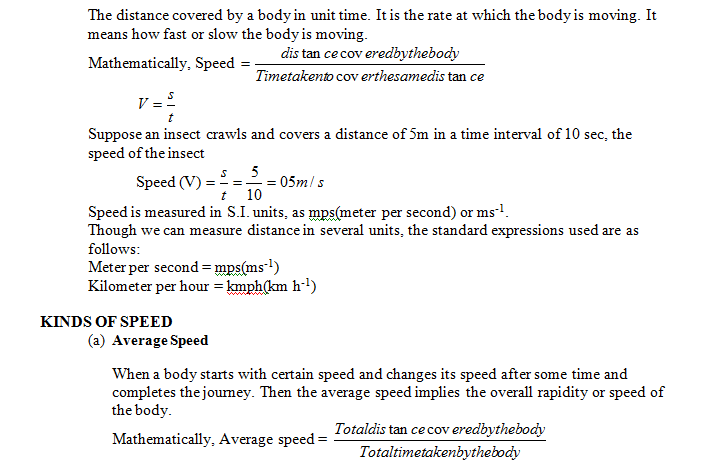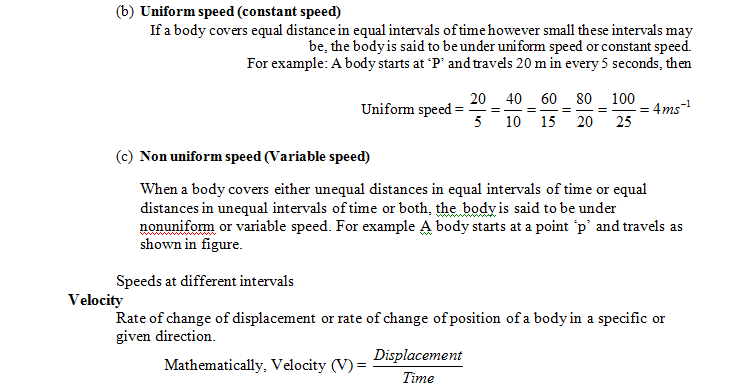# Distance & Displacement

## Motion and Time of Class 7

### DISTANCE

Distance travelled by a body is the length of the path taken irrespective of the direction of motion. SI unit of distance travelled is meter (m).

### DISPLACEMENT

Displacement of a body is the shortest distance travelled in a direction from initial to final point. SI unit of displacement is the meter (m) while its CGS unit is centimeter (cm).

The following example will elucidate it. If your house is situated far away from your school, different paths (routes) you travel to reach the school constitute distances. On the other hand the straight line path (actual distance) between your house and school can be termed as displacement.

### Few more examples

• When a ball is thrown vertically up about 5m high and catches it again, the total distance the body travels is 10m and the total displacement is zero, as it returns to the same point.
• When a body starts at A and comes to another end at B on semi circle, of radius ‘r. The distance the body travels is  (semi perimeter) and the displacement is 2r (diameter).
• When the same body completes the entire circle and comes to ‘A’ again, the distance covered is 2 and the displacement is zero

### SPEEDVelocity has same magnitude as speed but only direction is different hence its units are same both in S.I. and CGS systems (ms-1, cm s-1)

If someone states that speed of a running boy is 2 ms-1, it is very clear that he covers 2 m in one second. Now the question arises in which direction he is running then only it makes a complete sense and meaning. Therefore, it can be stated he is running with 2m per second from say’A’ to ‘B’ or say eastwards.

### KINDS OF VELOCITY

• Uniform velocity: When a body covers equal distances in equal intervals of time in a specified direction, however small these time intervals may be, the body is said to be moving with uniform velocity.Imagine a car is moving along a straight road towards east, with a velocity 60 kmph.Here the uniform velocity impies per each minute it covers a distance of 1 km and for each second it covers a distance of 1/60thkm.A body will have a uniform velocity only, if:
• It  covers equal distances in equal intervals of time, i.e., the magnitude does not change.
• Its direction remains the same. If any of the two conditions is not fulfilled, then the body will not be moving with a uniform velocity, but with a variable velocity.
• Variable Velocity: When a body covers unequal distances in equal intervals of time in a specified direction or equal distances in equal intervals of time, but its direction changes then the body is said to be moving with a variable velocity.
The following figure explains variable velocity clearly.
Now imagine the same car is moving along a circular path with the same velocity, 60 kmph (16.67 ms-1). Here the magnitude of the velocity i.e., 60 kmph is constant but direction is continuously changing. In this case (on circular path) the car is only under uniform speed but not under uniform velocity.Hence, when the car is covering equal distances is equal intervals of time, in the circular path the car is moving with a variable velocity, as the direction changes continuously.
• Average Velocity: The average displacement of a body per unit time, when the body is actually moving with variable velocity, is called average velocity.